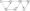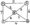# Analysis and Design of Algorithms July 2007

Note : Answer any FIVE full questions.

1 a. With the help of a flowchart, explain the various stages of algorithm design and analysis process.

b.  List the different problem types and name one algorithm of each type.

c.   Distinguish between the two common ways to represent a graph. Given the representation of undirected graph how do you ascertain that the graph is

i) Weighted          ii) Connected iii) Cyclic.

2 a. Explain in brief the basic asymptotic efficiency classes.

b.  Indicate whether the first function of each of the following pairs has a smaller, same or larger order of growth than second function

i) n(n+l) and 2000n2 ii) 100n2 and O.Oln3 iii) login and Inn

iv) 2n! and 2″ v) log2n and log2n2 vi) (n~ I)! and n

c.   Explain the concept of asymptotic notations indicating the normally used notations.

3 a. What is Brute force method? Write a Brute force string matching algorithm. Explain with suitable example the correctness of that algorithm. Analyse for complexity.

b.  List the sorting algorithms which use divide and conquer technique for sorting the elements. Write and analyse any one algorithm for sorting. Also give constraints of each algorithm.

4 a. Differentiate between depth first search and breadth first search techniques.

b.  What are the basic differences in representing the directed and undirected graph? Apply the depth first search based algorithm to solve the topological sorting problem for the following diagraph.c. Write the Johnson Trotter algorithm for generating permutation of the given set of numbers. Also generate all permutations of {3, 5, 7} by :

i)   The bottom up minimal change algorithm

ii)   The Johnson Trotter algorithm

iii)  The lexicographic order algorithm.

5 (a)Explain “Transform and Conquer” technique. What are the 3 major variations of this idea?

(b) The time efficiency of searching, inserting and deleting in a binary search tree is 0(log n) in the average case. It degenerates to 0(n) in the worst case. How is it overcome using transform and conquer technique? Explain both techniques by re-arranging the given data i)  7,8,10,5,4,6,9

ii) C, O, M, P, U, T, I, N, G

(C) Write and analyse the Horspool matching algorithm for searching a given pattern in a string.

6 (a) Write and explain the functions of Warshall’s and Floyd’s algorithm.

(b) Explain how the drawbacks of top-down and bottom-up approach of dynamic programming are overcome using memory functions. Apply the memory function

 Item Weight Value 1 3 \$25 2 2 \$20 3 1 \$15 Capacity W = 6 4 4 \$40 5 5 \$50

i) Indicate the entries of the dynamic programming table that are never computed by the memory function method on this instance.

7 (a) What is greedy technique? Explain how the different steps of this technique are taken care in generating a minimum spanning tree through a sequence of expanding subtrees. Apply this algorithm to the following graph.b.  Write KruskaPs algorithm. What are its applications? How is it different from prim’s algorithm?

 Character A B C D 1 Probability 0.4 0.1 0.2 0.15 0.15 1

Using this code encode the code ABCD-ABAC

8 a. Distinguish between P, NP and NP complete problems. Give examples for each category..

b.  Apply backtracking to solve the following instance of the subset sum problem

S = { 2, 3, 4, 5 } and d = 11 Will the backtracking algorithm work correctly if we use just one of the two in equalities to terminate a node as non-promising.

c.   Write short notes on :

i) Backtracking

ii)  Branch and bound technique.